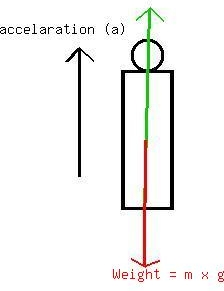Question# A person weighing 490 N stands on a scale in an elevator. The elevator is moving upward with an acceleration of 3m/s^2. The reading on the scale is the answer is 250 N. But i ended up with 340 N?

Other
ANSWEREDA person weighing 490 N stands on a scale in an elevator. The elevator is moving upward with an acceleration of $$\displaystyle{3}\frac{{m}}{{s}^{{2}}}$$. The reading on the scale is the answer is 250 N. But i ended up with 340 N?2020-11-04Green indicate normal force
First you have to clearly state what forces involve by free-body diagram (look above) applying Newton's law :
$$\displaystyle{F}={m}\times{a}$$
$$\displaystyle{\left({N}-{W}\right)}={m}\times{a}$$ then we have $$\displaystyle{N}={W}\times{m}{a}={m}{g}+{m}{a}={m}{\left({g}+{a}\right)}$$
where mass $$\displaystyle={\frac{{{W}}}{{{g}}}}={\frac{{{490}}}{{{9.8}}}}={50}\ {k}{g}$$
$$\displaystyle{N}={50}{\left({9.8}+{3}\right)}={50}{\left({12.3}\right)}={615}\ {N}$$